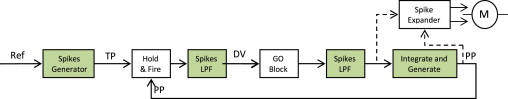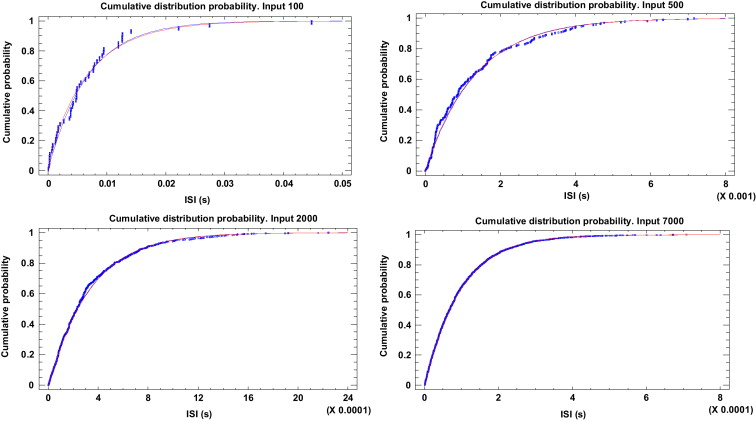# Inter-spikes-intervals exponential and gamma distributions study of neuron firing rate for SVITE motor control model on FPGA

Abstract: This paper presents a statistical study on a neuro-inspired spike-based implementation of the Vector-Integration-To-End-Point motor controller (SVITE) and compares its deterministic neuron-model stream of spikes with a proposed modification that converts the model, and thus the controller, in a Poisson like spike stream distribution. A set of hardware pseudo-random numbers generators, based on a Linear Feedback Shift Register (LFSR), have been introduced in the neuron-model so that they reach a closer biological neuron behavior. To validate the new neuron-model behavior a comparison between the Inter-Spikes-Interval empirical data and the Exponential and Gamma distributions has been carried out using the Kolmogorov–Smirnoff test. An in-hardware validation of the controller has been performed in a Spartan6 FPGA to drive directly with spikes DC motors from robotics to study the behavior and viability of the modified controller with random components.
The results show that the original deterministic spikes distribution of the controller blocks can be swapped with Poisson distributions using 30-bit LFSRs. The comparative between the usable controlling signals such as the trajectory and the speed profile using a deterministic and the new controller show a standard deviation of 11.53 spikes/s and 3.86 spikes/s respectively. These rates do not affect our system because, within Pulse Frequency Modulation, in order to drive the motors, time length can be fixed to spread the spikes. Tuning this value, the slow rates could be filtered by the motor. Therefore, this SVITE neuro-inspired controller can be integrated within complex neuromorphic architectures with Poisson-like neurons.Fig. 1. Block diagram of the neurocontroller where the Spike-based Vector Integration To-End Point (SVITE) algorithm is included. All the information flows as spikes. The Hold & Fire block computes the subtraction of two spike streams: current commanded position and target position (reference in spike domain). Green blocks represent where pseudo-random behavior has been included using LFSR. (For interpretation of the references to color in this figure legend, the reader is referred to the web version of this article.)Fig. 4. Cumulative distributions function for gamma and exponential approaches when a 24 bit LFSR and constant digital input reference values of 100, 500, 2000 and 7000.